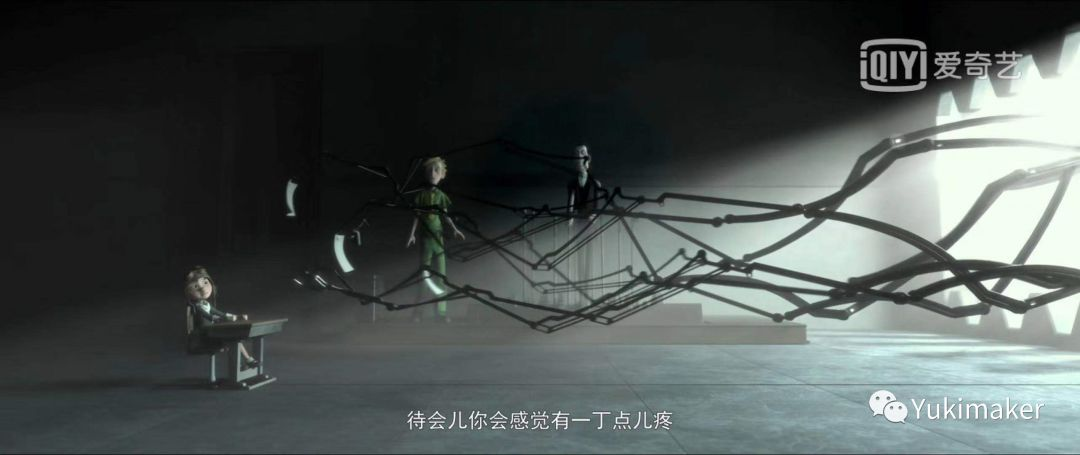武威信息网

# 透露着法式哲思的隽永童话' data-lazy='1' data-height='1463' width='828' height='auto'>' data-lazy='1' data-height='416' width='900' height='auto'>' data-lazy='1' data-height='379' width='900' height='auto'>' data-lazy='1' data-height='1080' width='828' height='auto'>' data-lazy='1' data-height='1777' width='828' height='auto'>' data-lazy='1' data-height='416' width='900' height='auto'>' data-lazy='1' data-height='732' width='828' height='auto'>“真正的问题从不在于长大，而在于遗忘。”' data-lazy='1' data-height='766' width='828' height='auto'>' data-lazy='1' data-height='1325' width='828' height='auto'>' data-lazy='1' data-height='1046' width='828' height='auto'>“你在她身上付出的时间使她无可代替”' data-lazy='1' data-height='1114' width='828' height='auto'>' data-lazy='1' data-height='416' width='900' height='auto'>' data-lazy='1' data-height='416' width='900' height='auto'>' data-lazy='1' data-height='379' width='900' height='auto'>' data-lazy='1' data-height='379' width='900' height='auto'>

“如果你想和别人制造牵绊，就要承受流泪的风险”

——